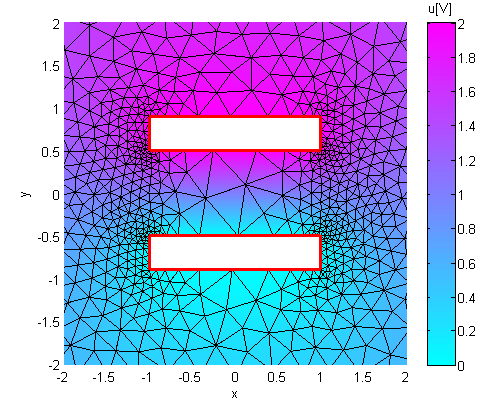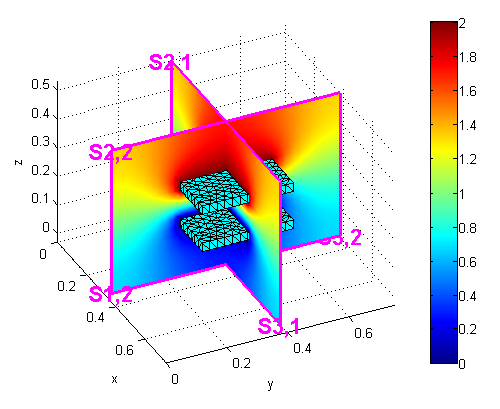FreeFEM Documentation on GitHub

stars - forks

# Plotting in Matlab and Octave

## Overview

In order to create a plot of a FreeFEM simulation in Matlab© or Octave two steps are necessary:

• The mesh, the finite element space connectivity and the simulation data must be exported into files

• The files must be imported into the Matlab / Octave workspace. Then the data can be visualized with the ffmatlib library

The steps are explained in more detail below using the example of a stripline capacitor.

Note

Finite element variables must be in P1 or P2. The simulation data can be 2D or 3D.

## 2D Problem

Consider a stripline capacitor problem which is also shown in Fig. 52. On the two boundaries (the electrodes) $$C_{A}$$, $$C_{K}$$ a Dirichlet condition and on the enclosure $$C_{B}$$ a Neumann condition is set. The electrostatic potential $$u$$ between the two electrodes is given by the Laplace equation

$\Delta u(x,y) = 0$

and the electrostatic field $$\mathbf{E}$$ is calculated by

$\mathbf{E} = -\nabla u$
 1 int CA=3, CK=4, CB=5;
2 real w2=1.0, h=0.4, d2=0.5;
3
4 border bottomA(t=-w2,w2){ x=t; y=d2; label=CA;};
5 border rightA(t=d2,d2+h){ x=w2; y=t; label=CA;};
6 border topA(t=w2,-w2){ x=t; y=d2+h; label=CA;};
7 border leftA(t=d2+h,d2){ x=-w2; y=t; label=CA;};
8
9 border bottomK(t=-w2,w2){ x=t; y=-d2-h; label=CK;};
10 border rightK(t=-d2-h,-d2){ x=w2; y=t; label=CK;};
11 border topK(t=w2,-w2){ x=t; y=-d2; label=CK;};
12 border leftK(t=-d2,-d2-h){ x=-w2; y=t; label=CK;};
13
14 border enclosure(t=0,2*pi){x=5*cos(t); y=5*sin(t); label=CB;}
15
16 int n=15;
17 mesh Th = buildmesh(enclosure(3*n)+
18              bottomA(-w2*n)+topA(-w2*n)+rightA(-h*n)+leftA(-h*n)+
19              bottomK(-w2*n)+topK(-w2*n)+rightK(-h*n)+leftK(-h*n));
20
21 fespace Vh(Th,P1);
22
23 Vh u,v;
24 real u0=2.0;
25
26 problem Laplace(u,v,solver=LU) =
27           int2d(Th)(dx(u)*dx(v) + dy(u)*dy(v))
28         + on(CA,u=u0)+on(CK,u=0);
29
30 real error=0.01;
31 for (int i=0;i<1;i++){
32    Laplace;
34    error=error/2.0;
35 }
36 Laplace;
37
38 Vh Ex, Ey;
39 Ex = -dx(u);
40 Ey = -dy(u);
41
42 plot(u,[Ex,Ey],wait=true);


## Exporting Data

The mesh is stored with the FreeFEM command savemesh(), while the connectivity of the finite element space and the simulation data are stored with the macro commands ffSaveVh() and ffSaveData(). These two commands are located in the ffmatlib.idp file which is included in the ffmatlib. Therefore, to export the stripline capacitor data the following statement sequence must be added to the FreeFEM code:

 1 include "ffmatlib.idp"
2
3 //Save mesh
4 savemesh(Th,"capacitor.msh");
5 //Save finite element space connectivity
6 ffSaveVh(Th,Vh,"capacitor_vh.txt");
7 //Save some scalar data
8 ffSaveData(u,"capacitor_potential.txt");
9 //Save a 2D vector field
10 ffSaveData2(Ex,Ey,"capacitor_field.txt");


## Importing Data

The mesh file can be loaded into the Matlab / Octave workspace using the ffreadmesh() command. A mesh file consists of three main sections:

1. The mesh points as nodal coordinates

2. A list of boundary edges including boundary labels

3. List of triangles defining the mesh in terms of connectivity

The three data sections mentioned are returned in the variables p, b and t. The finite element space connectivity and the simulation data can be loaded using the ffreaddata() command. Therefore, to load the example data the following statement sequence must be executed in Matlab / Octave:

 1 %Add ffmatlib to the search path
3 %Load the mesh
5 %Load the finite element space connectivity
7 %Load scalar data
9 %Load 2D vector field data


## 2D Plot Examples

ffpdeplot() is a plot solution for creating patch, contour, quiver, mesh, border, and region plots of 2D geometries. The basic syntax is:

1 [handles,varargout] = ffpdeplot(p,b,t,varargin)


varargin specifies parameter name / value pairs to control the plot behaviour. A table showing all options can be found in the ffmatlib documentation. A small selection of possible plot commands is given as follows:

• Plot of the boundary and the mesh:

1 ffpdeplot(p,b,t,'Mesh','on','Boundary','on');

• Patch plot (2D map or density plot) including mesh and boundary:

1 ffpdeplot(p,b,t,'VhSeq',vh,'XYData',u,'Mesh','on','Boundary','on', ...
2           'XLim',[-2 2],'YLim',[-2 2]);Fig. 53 Patch Plot with Mesh
• 3D surf plot:

1 ffpdeplot(p,b,t,'VhSeq',vh,'XYData',u,'ZStyle','continuous', ...
2           'Mesh','off');
3 lighting gouraud;
4 view([-47,24]);

• Contour (isovalue) and quiver (vector field) plot:

1 ffpdeplot(p,b,t,'VhSeq',vh,'XYData',u,'Mesh','off','Boundary','on', ...
2           'XLim',[-2 2],'YLim',[-2 2],'Contour','on','CColor','b', ...
3           'XYStyle','off', 'CGridParam',[150, 150],'ColorBar','off', ...
4           'FlowData',[Ex,Ey],'FGridParam',[24, 24]);Fig. 55 Contour and Quiver Plot

Matlab / Octave file

FreeFEM script

## 3D Plot Examples

3D problems are handled by the ffpdeplot3D() command, which works similarly to the ffpdeplot() command. In particular in three-dimensions cross sections of the solution can be created. The following example shows a cross-sectional problem of a three-dimensional parallel plate capacitor.Fig. 56 Slice on a 3D Parallel Plate Capacitor

Matlab / Octave file
FreeFEM script